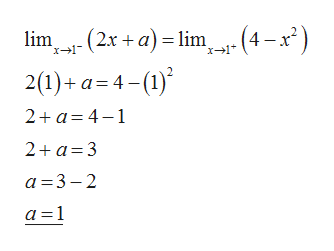# 1. (Find the value of a so that the function f(x) is continuous everywhere.f(x) ={2x+a if x<1    ;  4-x^2 if x>_ 1

Question
8 views

1. (Find the value of a so that the function f(x) is continuous everywhere.
f(x) ={2x+a if x<1    ;  4-x^2 if x>_ 1

check_circle

Step 1

Given:

Step 2

The function is continuous everywhere only when the right hand li...help_outlineImage Transcriptioncloselim .--(2.r+a)= lim "x1 - (4-x*) 2(1)+a 4-) 2+a 4-1 2+a 3 a 3-2 a 1 fullscreen

### Want to see the full answer?

See Solution

#### Want to see this answer and more?

Solutions are written by subject experts who are available 24/7. Questions are typically answered within 1 hour.*

See Solution
*Response times may vary by subject and question.
Tagged in

### Continuity